Question

# In any energy level, what is the maximum number electrons of a group of p-orbitals can...

In any energy level, what is the maximum number electrons of a group of p-orbitals can hold? (This is referring to all of the possible orientations when referring to the group, not just one orientation.) Your Answer: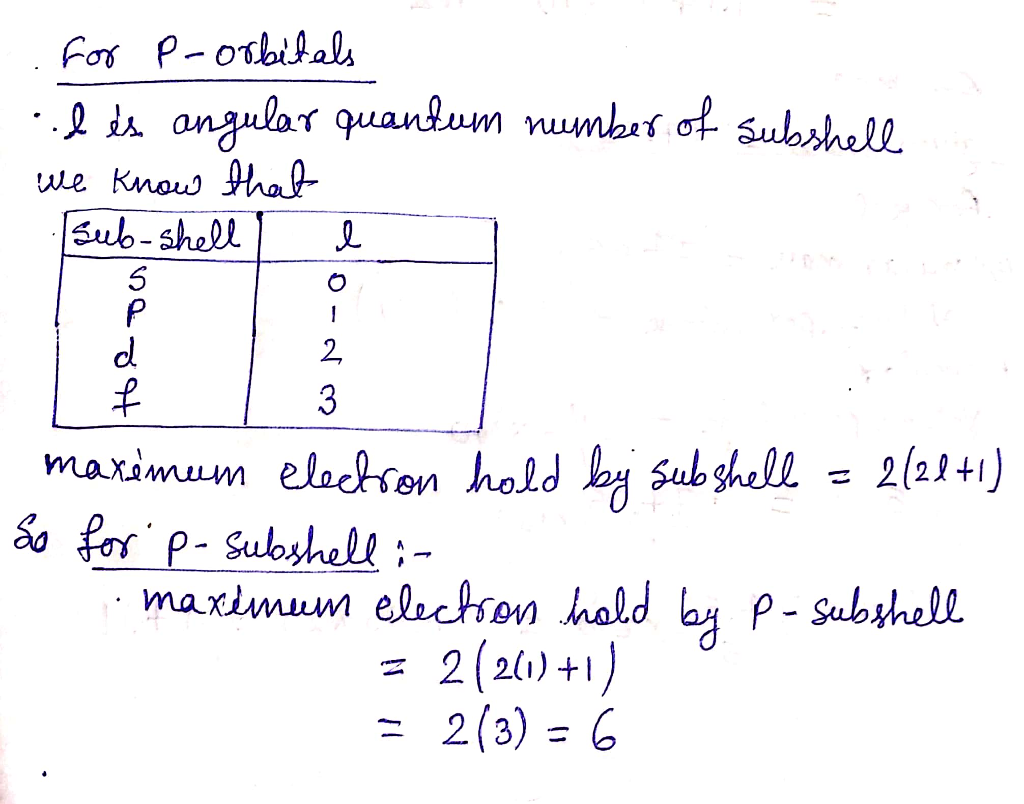Hence from the formula 2(2l+1) the maximum number of electron hold in p orbital is 6 election.

#### Earn Coins

Coins can be redeemed for fabulous gifts.

Similar Homework Help Questions
• ### 5) What is the maximum number of electrons that can occupy a 4f orbital? A) 2...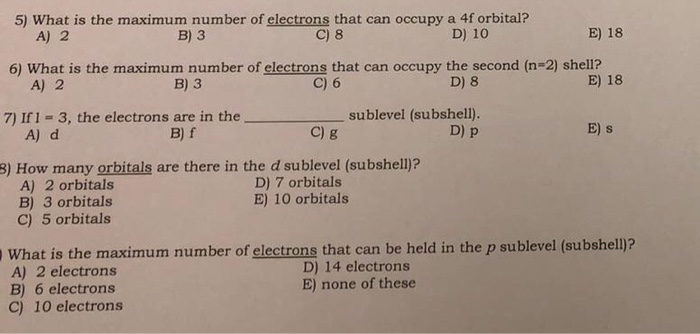5) What is the maximum number of electrons that can occupy a 4f orbital? A) 2 B) 3 C) 8 D) 10 E) 18 6) What is the maximum number of electrons that can occupy the second (n=2) shell? A) 2 B) 3 C) 6 D) 8 E) 18 7) If I = 3, the electrons are in the _sublevel (subshell). A) d B) f Dp E) s c) g 8) How many orbitals are there in the d sublevel...

• ### QUESTION 21 sublevl? f an energy level has t orbitals, how many are there in that...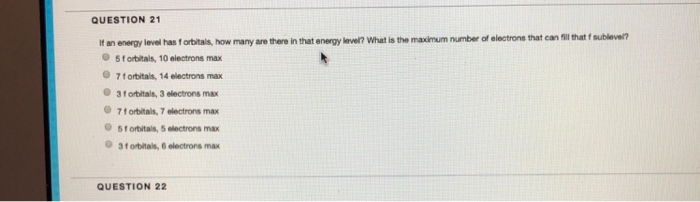QUESTION 21 sublevl? f an energy level has t orbitals, how many are there in that energy leve? What is the maximum number of electrons that can til hat f 5f orbitals, 10 electrons max 7fOrbitals, 14 electrons max 3f orbitals, 3 electrons max 7forbitals, 7 electrons max 5 f orbitals, 5 electrons max 3 t orbitals, 6 electrons max QUESTION 22

• ### 5. What is the maximum number of electrons in an atom that can have the following...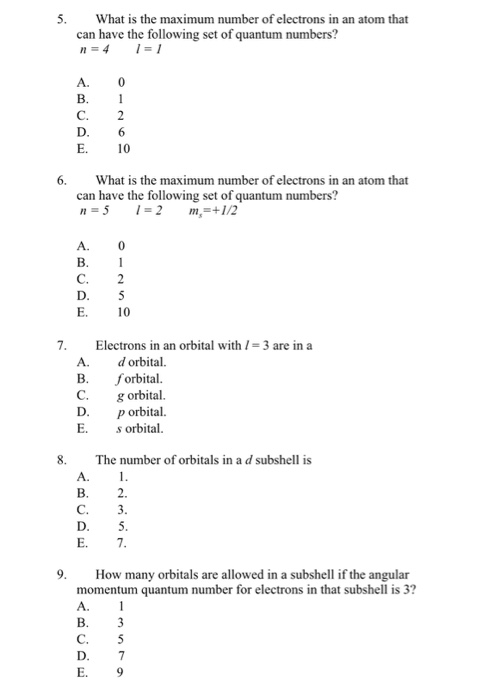5. What is the maximum number of electrons in an atom that can have the following set of quantum numbers? A. 0 C. 2 E. 10 6.What is the maximum number of electrons in an atom that can have the following set of quantum numbers? A. E. 10 7. Electrons in an orbital with3 are in a A. dorbital. В. forbital. C. orbital p orbital. E. orbital 8. The number of orbitals in a d subshell is E. 7 9....

• ### What is the total number of electrons that can occupy the 5f orbitals? List all the...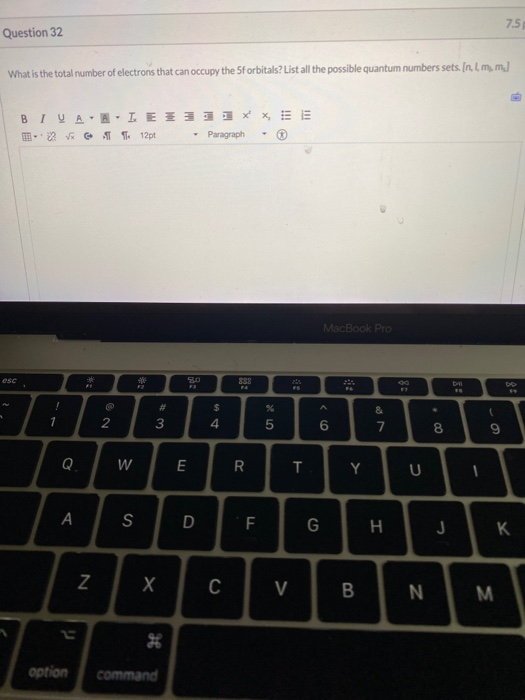What is the total number of electrons that can occupy the 5f orbitals? List all the possible quantum numbers sets [n,I,mI,ms] 75 Question 32 What is the total number of electrons that can occupy the forbitals? List all the possible quantum numbers sets. Inim, m.] E BIVAN IEE 2 T L 12pt * - Paragraph , - MacBook Pro oni w F option

• ### The maximum number of electrons that n, is can occupy an energy level described by the...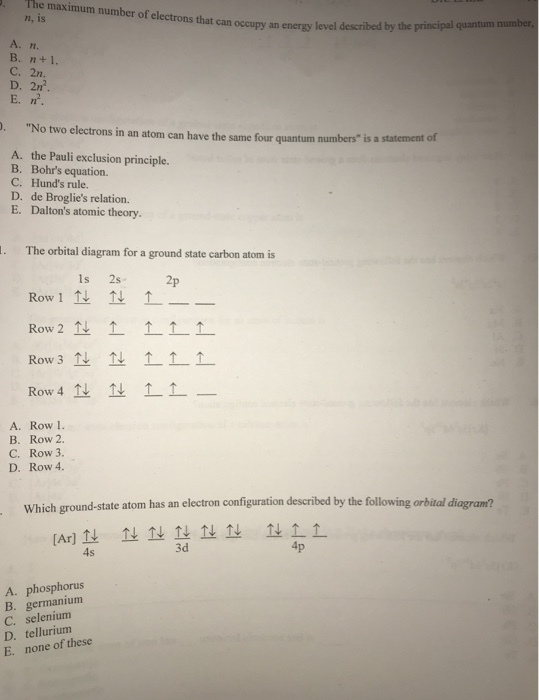The maximum number of electrons that n, is can occupy an energy level described by the principal quantum number an energy C. 2n D. 2n. . "No two electrons in an atom can have the same four quantum numbers is a statement of A. the Pauli exclusion principle B. Bohr's equation. C. Hund's rule. D. de Broglie's relation. E. Dalton's atomic theory. . The orbital diagram for a ground state carbon atom is 1s 2s 2p Row 1 ↑↓ ↑↓...

• ### In the animation, you can see that the electrons occupy different orbitals according to the energy...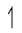In the animation, you can see that the electrons occupy different orbitals according to the energy level of each orbital. A single box represents an orbital. The unpaired electron is represented aswhereas the paired electrons in the same orbital are represented by two arrows pointing in opposite directions:. Watch the animation and identify which of the following statements are correct. Check all that apply. The He atom has two electrons that have parallel spin in its 1s orbital. In the...

• ### 4. (2) The 3rd main energy level (n=3) of atoms has 3 sublevel types designated by...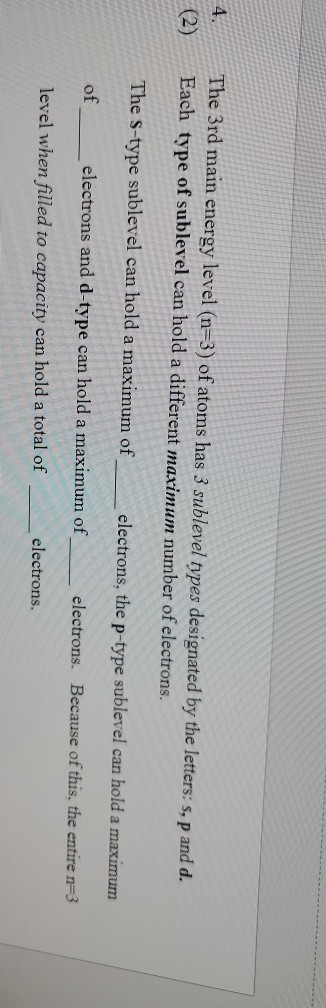4. (2) The 3rd main energy level (n=3) of atoms has 3 sublevel types designated by the letters: s,p and d. Each type of sublevel can hold a different maximum number of electrons. The S-type sublevel can hold a maximum of electrons, the p-type sublevel can hold a maximum of electrons and d-type can hold a maximum of electrons. Because of this, the entire n=3 level when filled to capacity can hold a total of electrons.

• ### What is the maximum number of electrons in an atom that can have the following quantum...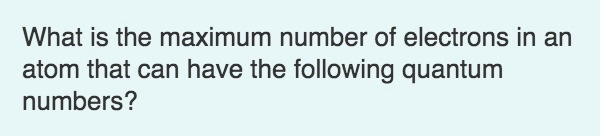What is the maximum number of electrons in an atom that can have the following quantum numbers? The quantum numbers n = 4,1 = 2, mı = 2 correspond to a particular orbital in the subshell. A single orbital can hold a maximum of electrons. two 4d 3s one three

• ### What is the maximum number of electrons in an atom whose highest-energy electrons have the principal...

What is the maximum number of electrons in an atom whose highest-energy electrons have the principal quantum number n=3? Express your answer as an integer. I've already tried putting in 18 and 28 electrons for my answer, but they were both marked as wrong. I'm not sure what else the answer could be.

• ### After the s, p, d, and f orbitals, the next higher series is the g series....

After the s, p, d, and f orbitals, the next higher series is the g series. a. How many electrons does each g subshell hold? b. What is the lowest principal energy level in which g orbitals can exist?